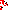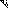# MINLPLib

### A Library of Mixed-Integer and Continuous Nonlinear Programming Instances

#### Instance nvs05

 Formatsⓘ ams gms mod nl osil py Primal Bounds (infeas ≤ 1e-08)ⓘ 5.47093411 p1 ( gdx sol ) (infeas: 2e-12) Other points (infeas > 1e-08)ⓘ Dual Boundsⓘ 5.47093410 (ANTIGONE)5.47093411 (BARON)5.47093411 (COUENNE)5.47093411 (LINDO)5.47093410 (SCIP)0.67402220 (SHOT) Referencesⓘ Gupta, Omprakash K and Ravindran, A, Branch and Bound Experiments in Convex Nonlinear Integer Programming, Management Science, 13:12, 1985, 1533-1546.Tawarmalani, M and Sahinidis, N V, Exact Algorithms for Global Optimization of Mixed-Integer Nonlinear Programs. In Pardalos, Panos M and Romeijn, H Edwin, Eds, Handbook of Global Optimization - Volume 2: Heuristic Approaches, Kluwer Academic Publishers, 65-85.Tawarmalani, M and Sahinidis, N V, Convexification and Global Optimization in Continuous and Mixed-Integer Nonlinear Programming: Theory, Algorithms, Software, and Applications, Kluwer, 2002. Sourceⓘ BARON book instance gupta/gupta05 Added to libraryⓘ 25 Jul 2002 Problem typeⓘ MINLP #Variablesⓘ 8 #Binary Variablesⓘ 0 #Integer Variablesⓘ 2 #Nonlinear Variablesⓘ 8 #Nonlinear Binary Variablesⓘ 0 #Nonlinear Integer Variablesⓘ 2 Objective Senseⓘ min Objective typeⓘ polynomial Objective curvatureⓘ indefinite #Nonzeros in Objectiveⓘ 4 #Nonlinear Nonzeros in Objectiveⓘ 4 #Constraintsⓘ 9 #Linear Constraintsⓘ 1 #Quadratic Constraintsⓘ 0 #Polynomial Constraintsⓘ 0 #Signomial Constraintsⓘ 4 #General Nonlinear Constraintsⓘ 4 Operands in Gen. Nonlin. Functionsⓘ div mul power sqr sqrt Constraints curvatureⓘ indefinite #Nonzeros in Jacobianⓘ 26 #Nonlinear Nonzeros in Jacobianⓘ 20 #Nonzeros in (Upper-Left) Hessian of Lagrangianⓘ 30 #Nonzeros in Diagonal of Hessian of Lagrangianⓘ 8 #Blocks in Hessian of Lagrangianⓘ 2 Minimal blocksize in Hessian of Lagrangianⓘ 3 Maximal blocksize in Hessian of Lagrangianⓘ 5 Average blocksize in Hessian of Lagrangianⓘ 4.0 #Semicontinuitiesⓘ 0 #Nonlinear Semicontinuitiesⓘ 0 #SOS type 1ⓘ 0 #SOS type 2ⓘ 0 Minimal coefficientⓘ 2.0474e-02 Maximal coefficientⓘ 1.0000e+15 Infeasibility of initial pointⓘ 4.74e+05 Sparsity JacobianⓘSparsity Hessian of Lagrangianⓘ```\$offlisting
*
*  Equation counts
*      Total        E        G        L        N        X        C        B
*         10        5        5        0        0        0        0        0
*
*  Variable counts
*                   x        b        i      s1s      s2s       sc       si
*      Total     cont   binary  integer     sos1     sos2    scont     sint
*          9        7        0        2        0        0        0        0
*  FX      0
*
*  Nonzero counts
*      Total    const       NL      DLL
*         31        7       24        0
*
*  Solve m using MINLP minimizing objvar;

Variables  i1,i2,x3,x4,x5,x6,x7,x8,objvar;

Integer Variables  i1,i2;

Equations  e1,e2,e3,e4,e5,e6,e7,e8,e9,e10;

e1.. -4243.28147100424/(x3*x4) + x5 =E= 0;

e2.. -sqrt(0.25*sqr(x4) + sqr(0.5*i1 + 0.5*x3)) + x7 =E= 0;

e3.. -(59405.9405940594 + 2121.64073550212*x4)*x7/(x3*x4*(0.0833333333333333*
sqr(x4) + sqr(0.5*i1 + 0.5*x3))) + x6 =E= 0;

e4.. -0.5*x4/x7 + x8 =E= 0;

e5.. -sqrt(sqr(x5) + 2*x5*x6*x8 + sqr(x6)) =G= -13600;

e6.. -504000/(sqr(i1)*i2) =G= -30000;

e7..    i2 - x3 =G= 0;

e8.. 0.0204744897959184*sqrt(1e15*POWER(i2,3)*i1*i1*POWER(i2,3))*(1 -
0.0282346219657891*i1) =G= 6000;

e9.. -0.21952/(POWER(i1,3)*i2) =G= -0.25;

e10.. -(1.10471*sqr(x3)*x4 + 0.04811*i1*i2*(14 + x4)) + objvar =E= 0;

* set non-default bounds
i1.lo = 1; i1.up = 200;
i2.lo = 1; i2.up = 200;
x3.lo = 0.01; x3.up = 200;
x4.lo = 0.01; x4.up = 200;

* set non-default levels
x3.l = 1;
x4.l = 1;
x5.l = 1;
x6.l = 1;
x7.l = 2;
x8.l = 1;

Model m / all /;

m.limrow=0; m.limcol=0;
m.tolproj=0.0;

\$if NOT '%gams.u1%' == '' \$include '%gams.u1%'

\$if not set MINLP \$set MINLP MINLP
Solve m using %MINLP% minimizing objvar;

```

Last updated: 2023-06-01 Git hash: fdbb0df7